# 时间复杂度到底怎么算

2019/04/10 10:10

• 时间维度：是指执行当前算法所消耗的时间，我们通常用「时间复杂度」来描述。
• 空间维度：是指执行当前算法需要占用多少内存空间，我们通常用「空间复杂度」来描述。

## 时间复杂度

• 常数阶$O(1)$
• 线性阶$O(n)$
• 平方阶$O(n^2)$
• 立方阶$O(n^3)$
• 对数阶$O(logn)$
• 线性对数阶$O(nlogn)$
• 指数阶$O(2^n)$

#### 常数阶$O(1)$

$O(1)$，表示该算法的执行时间（或执行时占用空间）总是为一个常量，不论输入的数据集是大是小，只要是没有循环等复杂结构，那这个代码的时间复杂度就都是O(1)，如：

int i = 1;
int j = 2;
int k = i + j;


#### 线性阶$O(n)$

$O(n)$，表示一个算法的性能会随着输入数据的大小变化而线性变化，如

for (int i = 0; i < n; i++) {
j = i;
j++;
}


#### 平方阶$O(n^2)$

$O(n²)$ 表示一个算法的性能将会随着输入数据的增长而呈现出二次增长。最常见的就是对输入数据进行嵌套循环。如果嵌套层级不断深入的话，算法的性能将会变为立方阶$O(n^3)$，$O(n^4)$，$O(n^k)$以此类推

for(x=1; i<=n; x++){
for(i=1; i<=n; i++){
j = i;
j++;
}
}


#### 指数阶$O(2^n)$

$O(2^n)$，表示一个算法的性能会随着输入数据的每次增加而增大两倍，典型的方法就是裴波那契数列的递归计算实现

int Fibonacci(int number)
{
if (number <= 1) return number;

return Fibonacci(number - 2) + Fibonacci(number - 1);
}


#### 对数阶$O(logn)$

int i = 1;
while(i<n)
{
i = i * 2;
}


#### 线性对数阶$O(nlogn)$

for(m=1; m<n; m++)
{
i = 1;
while(i<n)
{
i = i * 2;
}
}


## 空间复杂度

#### 空间复杂度 $O(1)$

int i = 1;
int j = 2;
++i;
j++;
int m = i + j;


#### 空间复杂度 $O(n)$

int[] m = new int[n]
for(i=1; i<=n; ++i)
{
j = i;
j++;
}


## 复杂度速查表

#### 图例#### 大-O 复杂度曲线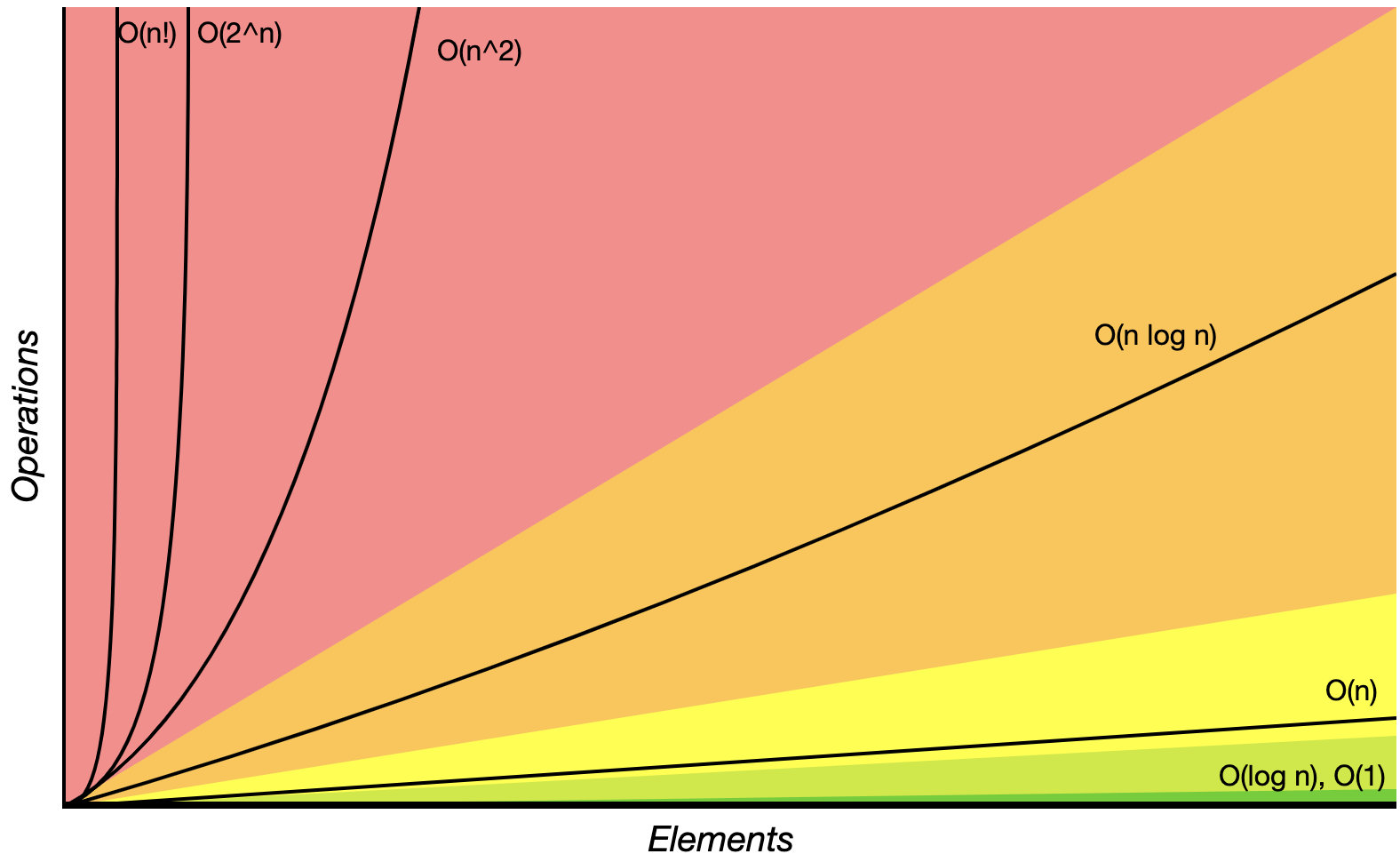#### 抽象数据结构的操作复杂度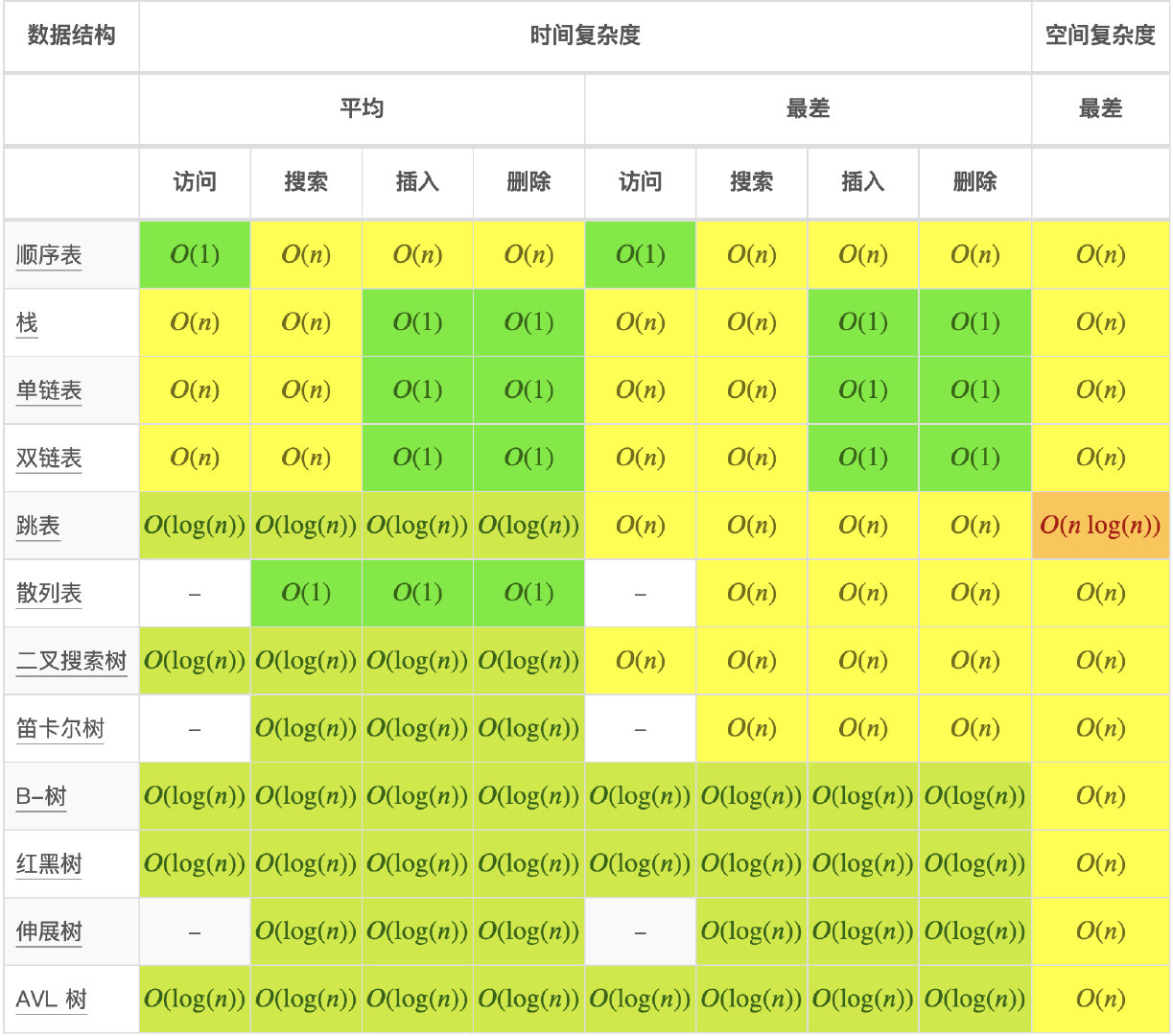#### 数组排序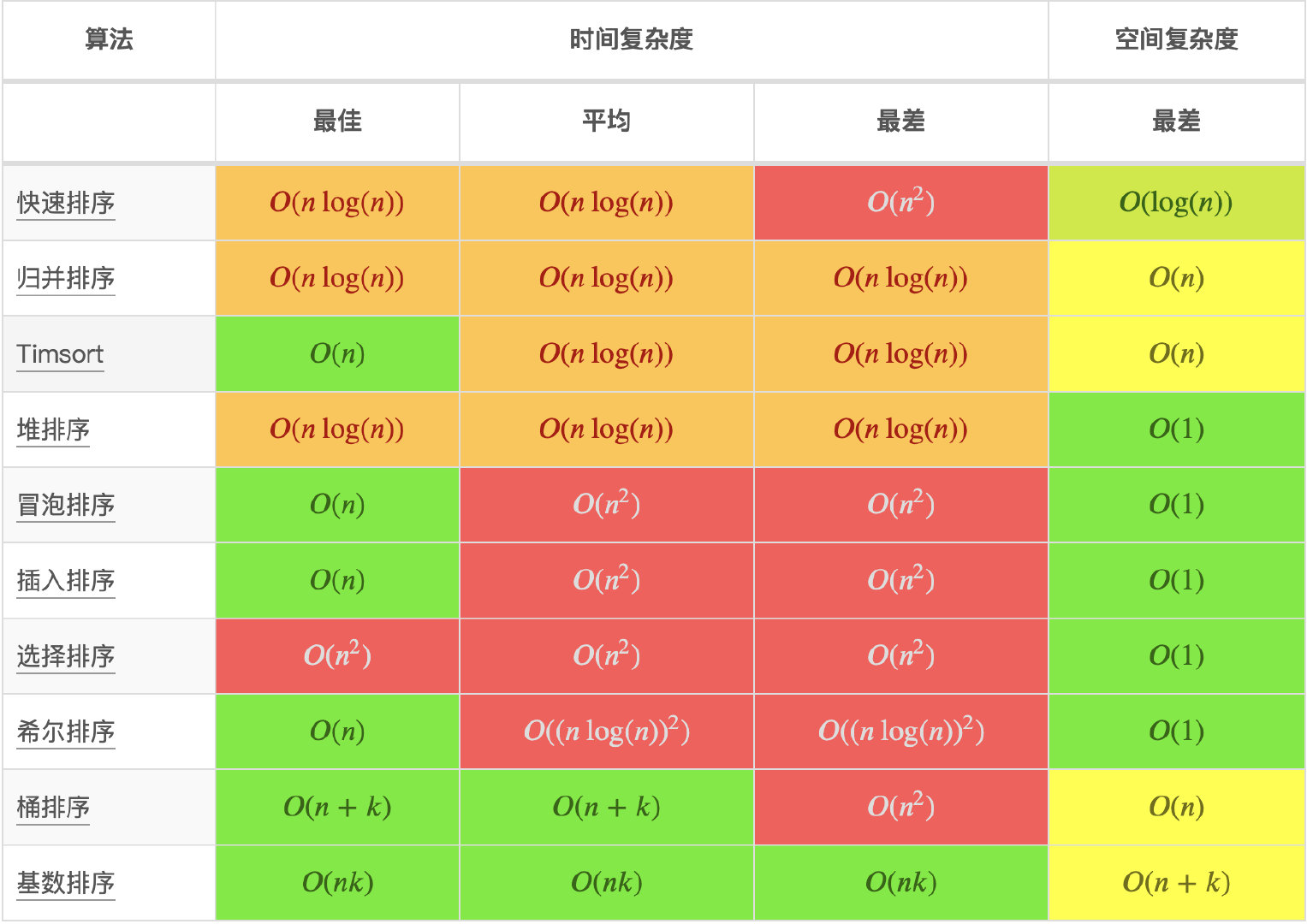#### 图操作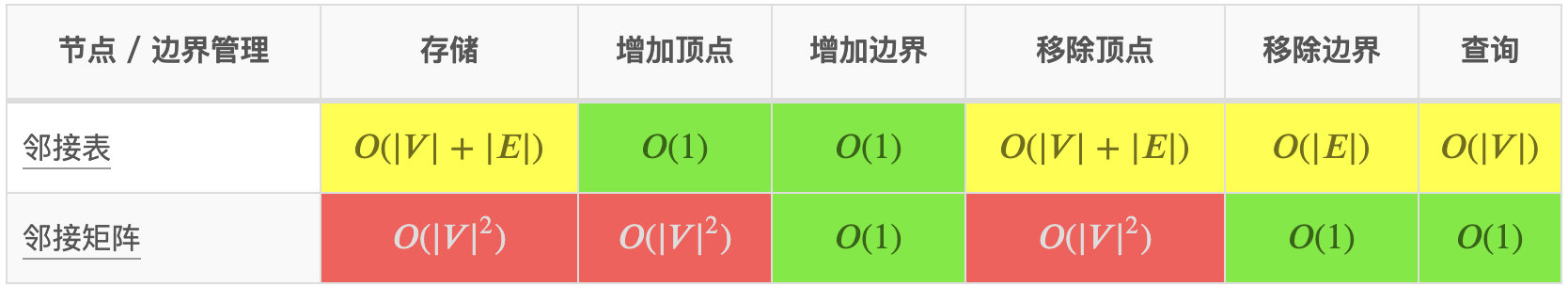#### 堆操作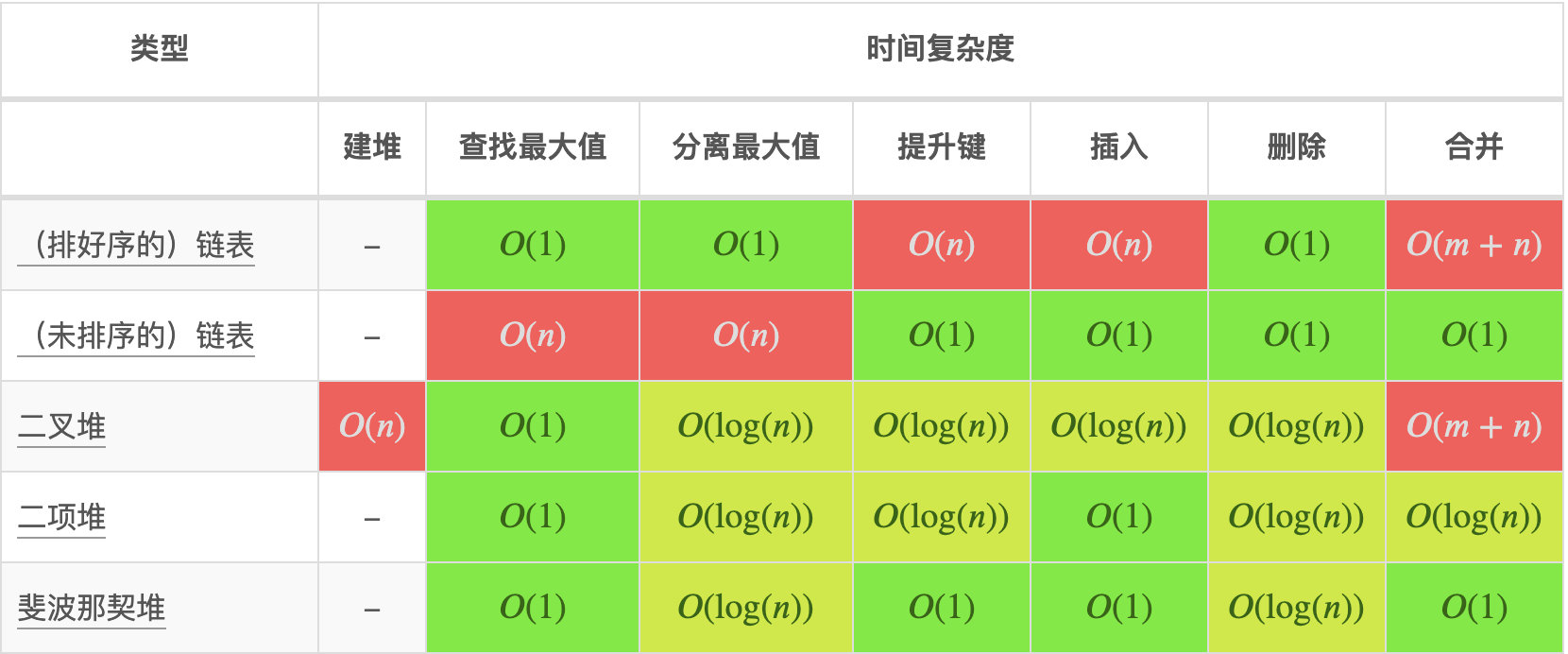## 参考### 作者的其它热门文章

0
0 收藏

0 评论
0 收藏
0